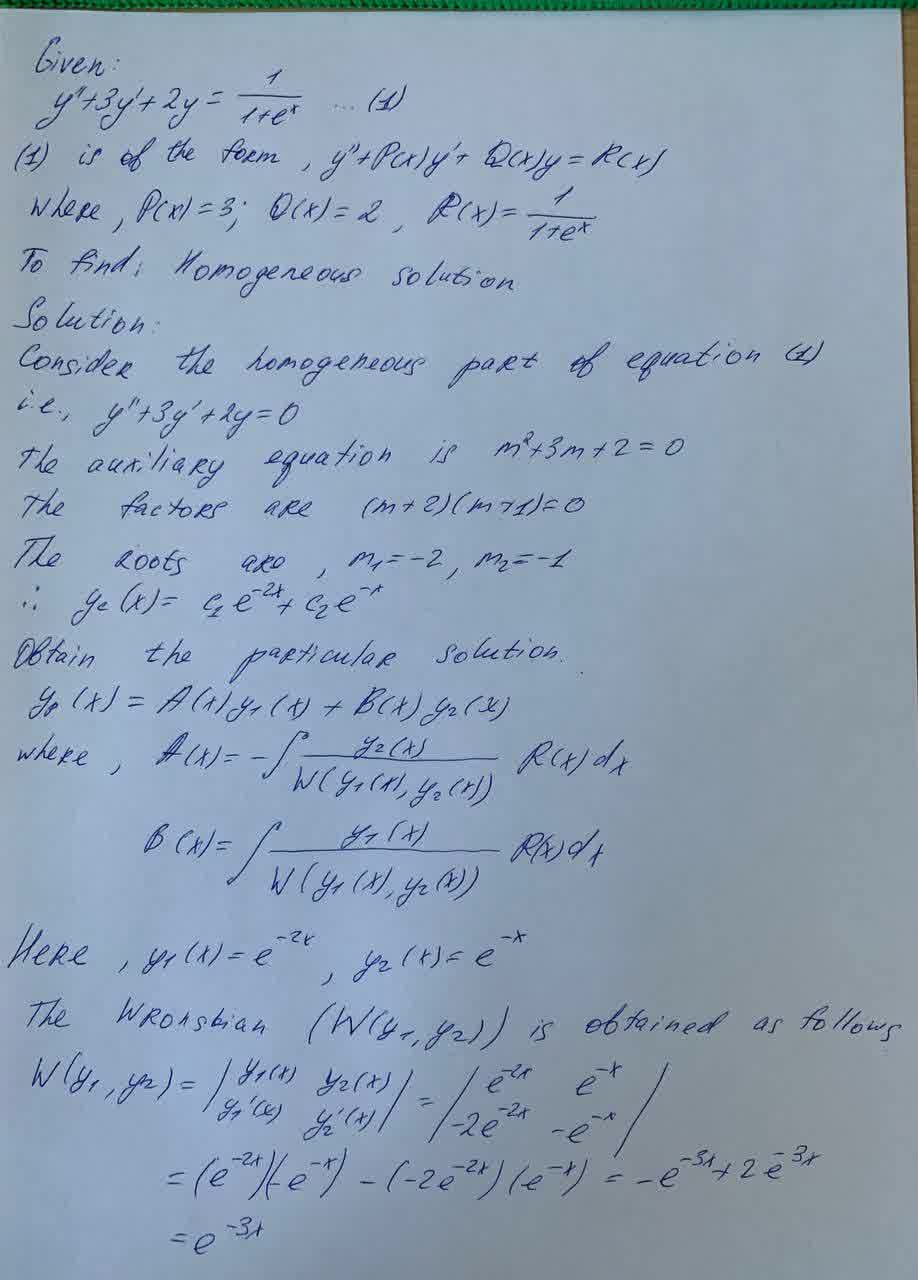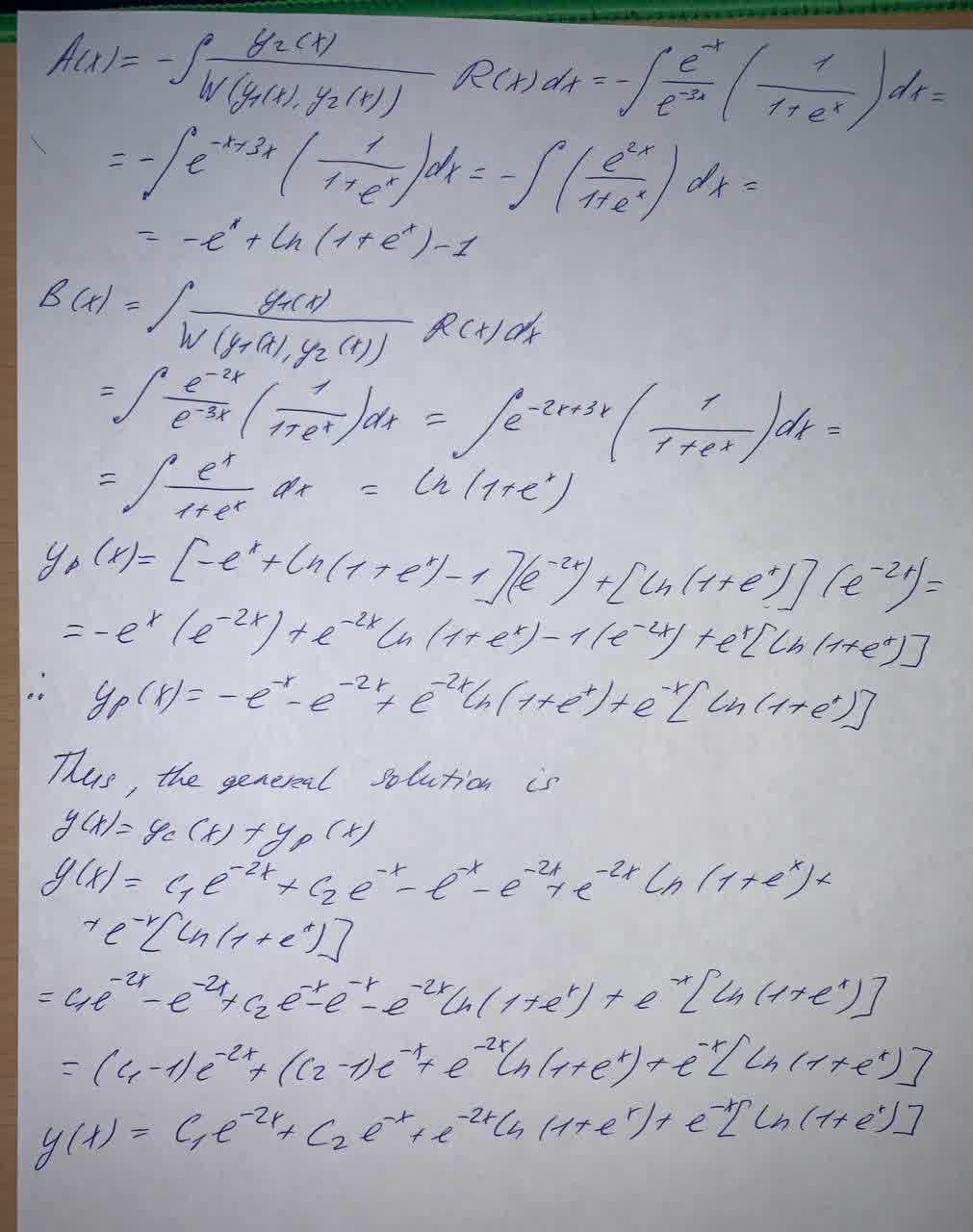Question# Solve the differential equation by variation of parameters y" + 3y' +2y = \frac{1}{1+e^x}

Differential equations
ANSWEREDSolve the differential equation by variation of parameters
$$y" + 3y' +2y = \frac{1}{1+e^x}$$